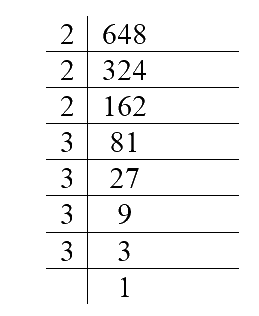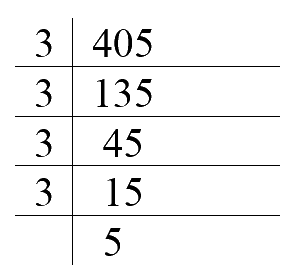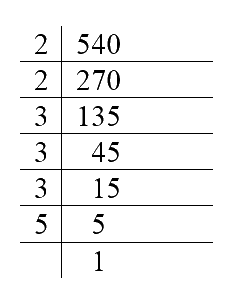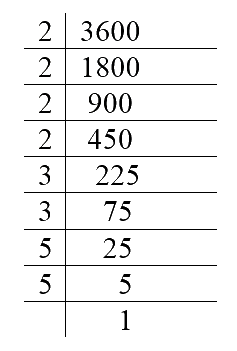NCERT Class 7 Maths Exponents and Powers

# NCERT Class 7 Maths Exponents and Powers

The chapter 13 begins with an introduction to exponents and powers by citing the example of the mass of the earth.Then, the concept of exponents is explained in detail.This is followed by the laws of exponents and the sub-topics under it include multiplying powers with the same base,dividing powers with the same base, taking power of a power, multiplying and dividing powers with the same exponents, and lastly numbers with exponents zero.Some miscellaneous examples using the Laws of Exponents id dealt in the next section.Decimal Number System and expressing large numbers in the standard form is the last topic of discussion.

## Chapter 13 Ex.13.1 Question 1

Find the value of:

(i) $$2^6$$

(ii) $$9^3$$

(iii) $$11^2$$

(iv) $$5^4$$

### Solution

What is known?.

Base and power.

What is unknown?.

Value of the given exponents.

Steps:

A larger number can be written in short form using exponential form in which there is a base and a smaller raised number called its power or exponent. Exponent represents how many times base will be multiplied. For example, $$64$$ can be written as $$2^6$$ here $$2$$ is base and $$6$$ is the exponent. $$2^6$$ means that $$2$$ is multiplied $$6$$ times $$8^2$$ means $$8$$ is multiplied $$2$$ times.

(i)

$${{2}^{6}}=2\times 2\times 2\times 2\times 2\times 2=64$$

(ii)

$${{9}^{3}}=9\times 9\times \ 9=729$$

(iii)

$${{11}^{2}}=11\times 11=121$$

(iv)

$${{5}^{4}}=5\times 5\times 5\times 5=625$$

## Chapter 13 Ex.13.1 Question 2

Express the following in exponential form:

(i) $$6\times 6\times 6\times 6$$

(ii) $$t \times t$$

(iii) $$b \times b \times b \times b$$

(iv) $$5\times 5\times 7\times 7\times 7$$

(v) $$2\times 2\times a \times a$$

(vi)  $$a \times a \times a \times c \times c \times c \times c \times d$$

### Solution

What is known?

Base of exponent.

What is unknown?

Power of the base.

Reasoning:

In this question a number is multiplied number of times and we have to express it in exponential form. As we know exponent is the number of times base is multiplied. So, will find the exponent and write it in exponential form.

(i) $$6\times 6\times 6\times 6$$

$$6$$ is multiplied $$4$$ times

Base $$=$$ $$6$$ and Exponent $$=$$ $$4$$

So, exponential form is $$6^4$$

(ii) $$t \times t$$

$$t$$ is multiplied $$2$$ times

Base $$=$$ $$t$$ and Exponent $$=$$ $$2$$

So, exponential form is $$=$$ $$t^2$$

(iii) $$b \times b \times b \times b$$

$$b$$ is multiplied $$4$$ times.

Base $$=$$ $$b,$$ exponent $$=$$$$4$$

So, exponential form is $$b^4$$

(iv) $$5\times 5\times 7\times 7\times 7$$

$$5$$ is multiplied $$2$$ times and $$7$$ is multiplied 3 times.

Base is $$5$$ and $$7$$ and exponent of $$5$$ is $$2$$ and $$7$$ is $$3.$$

So, exponential form is $$=$$ $$5^2\times$$  $$7^3$$

(v) $$2\times 2\times \text{a}\times \text{a}$$

$$2$$ is multiplied $$2$$ times, a is multiplied $$2$$ times.

Base is $$2$$ and $$a$$ and exponent of $$2$$ is $$2$$ and $$a$$ is $$2$$

So, exponential form is $$=$$ $$2^2 \times a^2$$  

(vi) $$a \times a \times a \times c \times c \times c \times c \times d$$

In this,$$a$$ is multiplied $$3$$ times, $$c$$ is multiplied $$4$$ times and $$d$$ once.

Base is $$a$$$$c$$ and $$d$$. Exponent of $$a$$ is $$3$$, $$c$$ is $$4$$ and $$d$$ is $$1$$

So,exponential form is $$a^3\times c^4 \times d$$

## Chapter 13 Ex.13.1 Question 3

Express each of the following numbers using exponential notation:

(i) $$512$$

(ii) $$343$$

(iii) $$729$$

(iv) $$3125$$

### Solution

What is known?

Numbers

What is unknown?

Base and Exponent of the given numbers.

Reasoning.

In this question, first find factors of the given number and then form its exponential form.

Steps:

(i) $$512$$

Factors$$= \left( \begin{array}{l}2 \times 2 \times 2 \times 2 \times 2 \times \\2 \times 2 \times 2 \times 2 \end{array} \right)$$

Base $$=$$ $$2$$ and exponent $$=$$ $$9$$

So, exponential form is $$2^9$$

(ii) $$343$$

Factors $$= 7 \times 7 \times 7$$

Base $$=$$ $$7$$ and exponent $$=$$ $$3$$

So, exponential form is $$=$$ $$7^3$$

(iii) $$729$$

Factors $$= 3 \times 3 \times 3 \times 3 \times 3 \times 3$$

Base $$=$$ $$3$$ and exponent $$=$$ $$6$$

So, exponential form $$3^6$$

(iv) $$3125$$

Factor$$= 5 \times 5 \times 5 \times 5 \times 5$$

Base $$=$$ $$5$$ and exponent $$=$$ $$5$$

So, exponential form $$5^5$$

## Chapter 13 Ex.13.1 Question 4

Identify the greater number, wherever possible, in each of the following?

(i) $${{4}^{3}}\text{ or }{{3}^{4}}\,$$

(ii) $${{5}^{3}}\text{ or }{{3}^{5}}$$

(iii) $${{2}^{8}}\text{ or }{{8}^{2}}$$

(iv) $${{100}^{2}}\text{ or }{{2}^{100}}$$

(v)  $${{2}^{10}}\text{ or }{{10}^{2}}$$

### Solution

What is known?

Exponential form

Reasoning:

In this question exponential form of the number is given. That means we know the base and exponent. We will solve by multiplying the given base to the number of times of its exponent and then compare the two number to find which is greater.

(i) $$4^3$$ or $$3^4$$

$$3$$ times $$4$$ $$=$$ $$4 \times 4 \times 4 =64$$

$$4$$ times $$3$$ $$=$$ $$\rm 3\times3\times3\times3 =81$$

Since $$81 \gt 64$$

So, $$3^4$$ is greater than $$4^3$$

(ii) $$5^3$$ or $$3^5$$

$$3$$ times $$5$$ $$=$$ $$\rm 5\times5\times5=125$$

$$5$$ times $$3$$ $$=$$ $$\rm 3\times3\times3\times3\times3=243$$

Since $$243 \gt 125$$

So, $$3^5$$ is greater than $$5^3$$

(iii) $$2^8$$ or $$8^2$$

$$8$$ times $$2$$ $$=$$ $$\rm 8\times8=256$$

$$2$$ times $$8$$ $$=$$ $$\left[ \begin{array}{l}{\rm{2}} \times {\rm{2}} \times {\rm{2}} \times {\rm{2}} \times \\{\rm{2}} \times {\rm{2}} \times {\rm{2}} \times {\rm{2}}\end{array} \right] = {\rm{64}}$$

Since $$256 \gt 64$$

So, $$2^8$$ is greater than $$8^2$$

(iv) $$100^2$$ or $$2$$$$100$$

$$2$$ times $$100$$ $$=$$ $$\rm 100 \times 100 =10,000$$

$$100$$ times $$\left[ \begin{array}{l}{\rm{2 = 2}} \times {\rm{2}} \times {\rm{2}} \times {\rm{2}} \times \\{\rm{2}} \times ........ \times {\rm{100}}\end{array} \right]$$ times $$= 16,384$$

Since $$16,384 > 10,000$$

So, $$100^2$$ is greater than $$2^{100}$$

(v) $$2$$$$10$$ or $$10^2$$

$$10$$ times $$2$$ $$=$$ $$1024$$

$$2$$ times $$10$$ $$=$$ $$100$$

Since $$1024 > 100$$

So, $$2$$$$10$$ is greater than $$10^2$$

## Chapter 13 Ex.13.1 Question 5

Express each of the following as product of powers of their prime factors:

(i) $$648$$

(ii) $$405$$

(iii) $$540$$

(iv) $$3,600$$

### Solution

What is known?

Numbers.

What is unknown?

Prime factors and powers of given numbers.

Reasoning:

In this question, first find prime factors of the given number and then raise the prime factor to its powers.

Steps:

(i) $$648$$Prime factorization of $$648$$ $$= 2 \times 2 \times 2 \times 3 \times 3 \times 3 \times 3$$

In this, $$3$$ is the exponent of $$2$$ and $$4$$ is the exponent of $$3$$

So, $$648$$ can be expressed as product of powers of their prime factors as $$2^3 \times$$$$3^4$$

(ii) $$405$$Prime factorization of $$405$$ $$= 3 \times3 \times 3 \times 3 \times 5$$

In this, $$4$$ is the exponent of $$3$$ and $$1$$ is the exponent of $$5$$

So, product of powers of prime factors $$=$$ $$3^3 \times$$ $$5$$

(iii) $$540$$Prime factorization of $$540$$ $$= 2 \times 2 \times 3 \times 3 \times 3 \times 5$$

In this, $$2$$ is the exponent of $$2$$. $$3$$ is the exponent of $$3$$ and $$1$$ is the exponent of $$5$$

So, product of powers of prime factors $$= 2^2 \times 3^3 \times 5$$

(iv) $$3,600$$Prime factorization of $$540$$ $$= \left[ \begin{array}{l}2 \times 2 \times 2 \times 2 \times \\3 \times 3 \times 5 \times 5\end{array} \right]$$

In this, $$4$$ is the exponent of $$2$$. $$2$$ is the exponent of $$3$$ and $$2$$ is the exponent of $$5$$

So, product of powers of prime factors $$= 2^4 \times 3^2 \times 5^2$$

## Chapter 13 Ex.13.1 Question 6

Simplify:

(i)  $$2\times {{10}^{3}}$$

(ii) $${{7}^{2}}\times {{2}^{2}}$$

(iii) $${{2}^{3}}\times 5$$

(iv) $$3\times {{4}^{4}}$$

(v) $$0\times {{10}^{2}}$$

(vi) $${{5}^{2}}\times {{3}^{3}}$$

(vii) $${{2}^{4}}\times {{3}^{2}}$$

(viii)  $${{3}^{2}}\times {{10}^{4}}$$

### Solution

What is known?

Base and exponent.

What is unknown?

Simple form of the number.

Reasoning:.

In this question we will multiply the base and the number of times it’s exponent to simplify the given statement.

(i)

\begin{align} 2\times {{10}^{3}}&=2\times (10\times 10\times 10)\\&=2\times 1000\\&=2000\end{align}

(ii)

\begin{align} {{7}^{2}}\times {{2}^{2}}&=(7\times 7)\times (2\times 2)\\&=49\times 4\\&=196\end{align}

(iii)

\begin{align}{{2}^{3}}\times 5&=(2\times 2\times 2)\times 5\\&=8\times 5\\&=40\end{align}

(iv)

\begin{align}3\times {{4}^{4}}&=3\times (4\times 4\times 4\times 4)\\&=3\times 256\\&=768\end{align}

(v)

\begin{align}0\times {{10}^{2}}&=0\times 10\times 10\\&=0\end{align}

(vi)

\begin{align}{{5}^{2}}\times {{3}^{3}}&=(5\times 5)\times (3\times 3\times 3)\\&=25\times 27\\&=675\end{align}

(vii)

\begin{align}{{2}^{4}}\!\times \!{{3}^{2}}&=(\!2\times\!\! 2\times \!2\times\!2)\!\times \!(3\!\times\!3)\\&=16\!\times\! 9\\&=144\end{align}

(viii)

\begin{align}{3^2}\!\! \times\!\! {10^4}&=\!\! (3\!\!\times\!\!3)\!\!\times\!\!(10\!\!\times\!\!10\!\!\times\!\!10\!\!\times\!\!10) \\&= 9\!\times\!10000 \\&= 90000\end{align}

## Chapter 13 Ex.13.1 Question 7

Simplify:

(i) $${{(-4)}^{3}}$$

(ii) $$(-3)\times {{(-2)}^{3}}$$

(iii) $${{(-3)}^{2}}\times {{(-5)}^{2}}$$

(iv)  $${{(-2)}^{3}}\times {{(-10)}^{3}}$$

### Solution

What is unknown?

Base and Exponent

Reasoning:

In this question we will multiply the base and the number of times it’s exponent to simplify the given statement.

Steps:

(i) $${{(-4)}^{3}}$$

\begin{align} &\!=\!-4\times\! -4\!\times\! -4\!\\&=\!-\!64 \end{align}

(ii) $$(-3)\times {{(-2)}^{3}}$$

\begin{align}&=\!-3x\left( -2\!\times\!\!\!-2\times\!\!-2 \right)\\&=-3\!\times\!\!-8\\&=24\end{align}

(iii) $${{(-3)}^{2}}\times {{(-5)}^{2}}$$

\begin{align}&\!=\!-3x\!-\!3x(\!-5x\!-5)\\&=9\times 25\\&=225 \end{align}

(iv)  $${{(-2)}^{3}}\times {{(-10)}^{3}}$$

\begin{align}&\!=\!-\!\!2x\!-\!\!2x\!-\!\!2x(\!-\!\!10x\!-\!\!10x\!-\!\!10)\\&=-8x-1000\\&=8000 \end{align}

## Chapter 13 Ex.13.1 Question 8

Compare the following numbers:

(i) $$2.7\times {{10}^{12}} ; \,k1.5\times {{10}^{8}}$$

(ii) $$4\times {{10}^{14}};3\times {{10}^{17}}$$

### Solution

What is known?

Two numbers with base $$10$$ but different powers.

What is unknown?

Out of the given two numbers, which number is greater or smaller.

Reasoning:

In this question, simplify the numbers and decide which one is greater. Another way is to look at the power of $$10$$. The number with higher power of $$10$$ is greater than the other.

Steps:

(i) In numbers,$$2.7 \! \times \! 10^{12}$$ and $$1.5 \! \times \! 10^8$$

\begin{align}2.7 \times 10^{12} &= 2.7 \times 12\,\rm{ times}\,\\10 &= 2.7 \times 1000000000000 \\&= 27,00,00,00,00,000\end{align}

And

\begin{align}1.5 \times 10^8 &= 1.5 \times 8\, \rm{times}\\10 &= 1.5 \times 100000000\\&= 15,00,00,000\end{align}

So, $$2.7 \! \times \! 10^{12}$$ is greater than $$1.5 \! \times \! 10^8$$

(ii) In numbers, $$4 \! \times \! 10^{14}; \;3 \! \times \! 10^{17}$$

\begin{align}4 \times 10^{14}&= 4 \times 14\,\rm{times} \\10&= 4 \times 100000000000000 \\&= 4,00,00,00,00,00,000\end{align}

And

\begin{align}3 \! \times \! 10^{17} &\!=\!3\!\times\!17\,\rm{ times }\\10 &\!=\! 3\! \times\! 100000000000000000 \\&\!=\! 3,00,00,00,00,00,00,00,000\end{align}

So, $$3 \! \times \! 10^{17}$$ is greater than $$4 \! \times \! 10^{14}$$

Exponents and Powers | NCERT Solutions
Instant doubt clearing with Cuemath Advanced Math Program